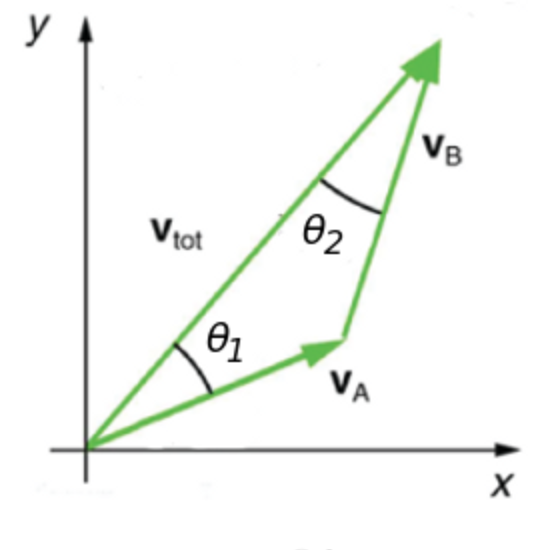# Problem: The figure depicts the sum of two velocities, vA and vB. The magnitude of the sum is 6.5 m/s and the angles shown in the image are θ1 = 29.8°, and θ2 = 21.8°. Find the magnitude of velocity VA and VB in meters per second.

###### FREE Expert Solution

In this problem, we'll use the sine rule in triangle.

87% (260 ratings)###### Problem Details

The figure depicts the sum of two velocities, vA and vB. The magnitude of the sum is 6.5 m/s and the angles shown in the image are θ1 = 29.8°, and θ2 = 21.8°. Find the magnitude of velocity VA and VB in meters per second.